• 数据统计条形图PPT图表素材下载，关键词：PPT图表，条形图，条状图，数据分析，数据统计，幻灯片素材； 数据统计条形图PPT图表素材下载，关键词：PPT图表，条形图，条状图，数据分析，数据统计，幻灯片素材；
• Python处处理理JSON数数据据并并生生成成条条形形图图 本文详细介绍如何利用Python进行数据分析尝试处理一份JSON数据并生成条形图的过程有需要的朋友们可以 参 学习 一一JSON 数数据据准准备 首先准备一份 JSON 数据...
• 本文实例为大家分享了python读取mysql数据绘制条形图的具体代码，供大家参考，具体内容如下 Mysql 脚本示例： create table demo( id int ,product varchar(50) ,price decimal(18,2) ,quantity int ,amount ...
• 数据统计条形图PPT图表素材下载，关键词：PPT图表，条形图，条状图，数据分析，数据统计，幻灯片素材；
• # -*- coding: utf-8 -*-from __future__ import unicode_literalsimport matplotlib.pyplot as mpimport numpy as npn = 12x = np.arange(n)y1 = np.random.uniform(0.5, 1.0, n) * (1 - x / n)y2 = np.random.unif...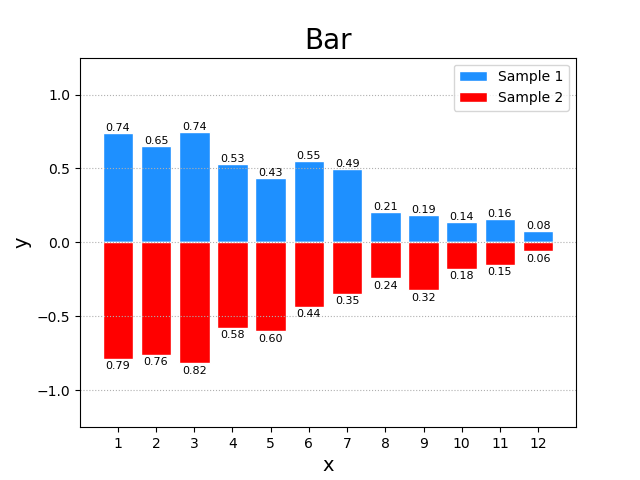# -*- coding: utf-8 -*-

from __future__ import unicode_literals
import matplotlib.pyplot as mp
import numpy as np

n = 12
x = np.arange(n)
y1 = np.random.uniform(0.5, 1.0, n) * (1 - x / n)
y2 = np.random.uniform(0.5, 1.0, n) * (1 - x / n)
mp.figure('Bar', facecolor='lightgray')
mp.title('Bar', fontsize=20)
mp.xlabel('x', fontsize=14)
mp.ylabel('y', fontsize=14)
mp.xticks(x, x + 1)
mp.ylim(-1.25, 1.25)
mp.tick_params(labelsize=10)
mp.grid(axis='y', linestyle=':')
mp.bar(x, y1, ec='white', fc='dodgerblue', label='Sample 1')
for _x, _y in zip(x, y1):
mp.text(_x, _y, '%.2f' % _y, ha='center', va='bottom', size=8)
mp.bar(x, -y2, ec='white', fc='red', label='Sample 2')
for _x, _y in zip(x, y2):
mp.text(_x, -_y - 0.015, '%.2f' % _y, ha='center', va='top', size=8)
mp.legend()
mp.show()自己练习的作品,欢迎交流
展开全文python
• 一、JSON 数据准备 首先准备一份 JSON 数据，这份数据共有 3560 条内容，每条内容结构如下： 本示例主要是以 tz（timezone 时区） 这一字段的值，分析这份数据里时区的分布情况。...生成条形图之前，为了数据的完整
• 假设你获取到了2017年内地电影票房前20的电影(列表a)和电影票房数据(列表b),那么如何更加直观的展示该数据? a = [“战狼2”,“速度与激情8”,“功夫瑜伽”,“西游伏妖篇”,“变形金刚5：最后的骑士”,“摔跤吧！爸爸...
• 这次接着介绍如何读取数据绘制条形图 读取csv中的年龄并绘制成区间图 只是一个很简单的实例，如果需要扩展丰富，只需要往这个模板往上加就是了 csv文件链接：https://pan.baidu.com/s/1aLlGEcZEkMiTf9Pfsh-9WQ  ...
之前介绍了如何读取csv数据绘制饼状图

这次接着介绍如何读取数据绘制条形图

读取csv中的年龄并绘制成区间图

只是一个很简单的实例，如果需要扩展丰富，只需要往这个模板往上加就是了
csv文件链接：https://pan.baidu.com/s/1aLlGEcZEkMiTf9Pfsh-9WQ
提取码：jr5t 

import csv
import matplotlib
import matplotlib.pyplot as plt
import numpy as np
import pandas as pd

def age_Dis():#统计年龄分布
print("统计年龄分布：")
print()
age = []
for i in pr['BIRTHDAY']:
age.append(int((20181219 - i) / 10000))
print(age)
age1=[]
age2=[]
age3=[]
age4=[]
age5=[]
for i in age:
if 18<=i<25:
age1.append(i)
elif 25<=i<35:
age2.append(i)
elif 35<=i<45:
age3.append(i)
elif 45<=i<55:
age4.append(i)
else:
age5.append(i)

index=['18~25','25~35','35~45','45~55','others']
values=[len(age1),len(age2),len(age3),len(age4),len(age5)]
plt.bar(index,values)
plt.show()

if __name__=="__main__":
age_Dis()

展示结果：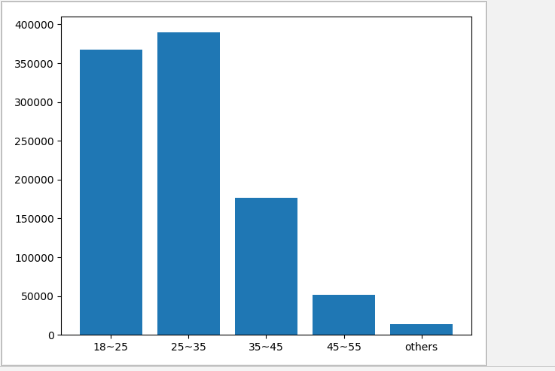注：

问：为什么要用条形图？？

答：数据太多（一百万多条），如果用其他图就不能很好的显示出来

比如说，使用折线图：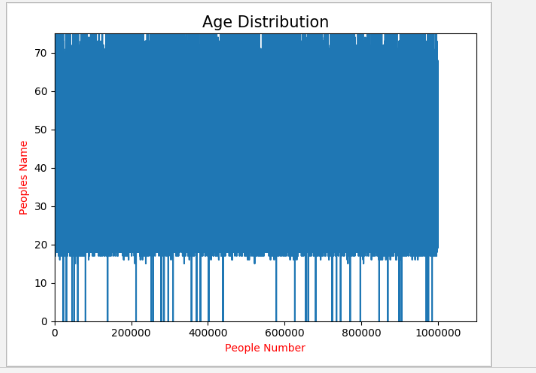如图：就完全看不出年龄的分布情况

代码如下：

# 绘制成折线图'''
'''
plt.axis([0, 1100000, 0, 75])
plt.xlabel('People Number',color='red')
plt.ylabel('Peoples Name',color='red')
plt.title('Age Distribution',fontsize=15)
plt.plot(age)
plt.show()
print("折线图效果太差，因采用柱形图")
'''

因而，在数据过大的情况下，最好采用条形图
展开全文python
• Python 读取mysql数据绘制条形图
Mysql 脚本示例：
create table demo(
id int
,product varchar(50)
,price decimal(18,2)
,quantity int
,amount decimal(18,2)
,orderdate datetime
);
insert into demo
select 1,'AAA',15.2,5,76,'2017-09-09' union all
select 2,'BBB',10,6,60,'2016-05-18' union all
select 3,'CCC',21,11,231,'2014-07-11' union all
select 4,'DDD',55,2,110,'2016-12-24' union all
select 5,'EEE',20,4,80,'2017-02-08' union all
select 6,'FFF',45,2,90,'2016-08-19' union all
select 7,'GGG',33,5,165,'2017-10-11' union all
select 8,'HHH',5,40,200,'2014-08-30' union all
select 9,'III',3,20,60,'2015-02-25' union all
select 10,'JJJ',10,15,150,'2015-11-02';python 绘图分析：
# -*- coding: utf-8 -*-
#import numpy
import MySQLdb
import plotly.plotly
import plotly.graph_objs as pg

host = "localhost"
port = 3306
user = "root"
passwd = "mysql"
charset = "utf8"
dbname = "test"
conn = None

try:
conn = MySQLdb.Connection(
host = host,
port = port,
user = user,
passwd = passwd,
db = dbname,
charset = charset
)
cur = conn.cursor(MySQLdb.cursors.DictCursor)
cur.execute("select * from demo;")
rows = cur.fetchall()
#rows = numpy.array(rows)
lists = [[],[],[],[]]
for row in rows:
lists.append(row["product"])
lists.append(row["price"])
lists.append(row["quantity"])
lists.append(row["amount"])
#print(lists)
#print(lists)
#print(([x for x in lists]))

date_price = pg.Bar( x=lists, y=lists, name='价格')
date_quantity = pg.Bar( x=lists, y=lists, name='数量')
date_amount = pg.Bar( x=lists, y=lists, name='总价')
data = [date_price, date_quantity, date_amount]
#barmode = [stack,group,overlay,relative]
layout = pg.Layout( barmode='group',title="各产品销售情况" )
fig = pg.Figure(data=data, layout=layout)
plotly.offline.plot(fig, filename = "C:/Users/huangzecheng/Desktop/test.html")

finally:
if conn:
conn.close()
将代码保存为文件
bartest.py ，执行脚本 python bartest.py ，生成 html 文件如下：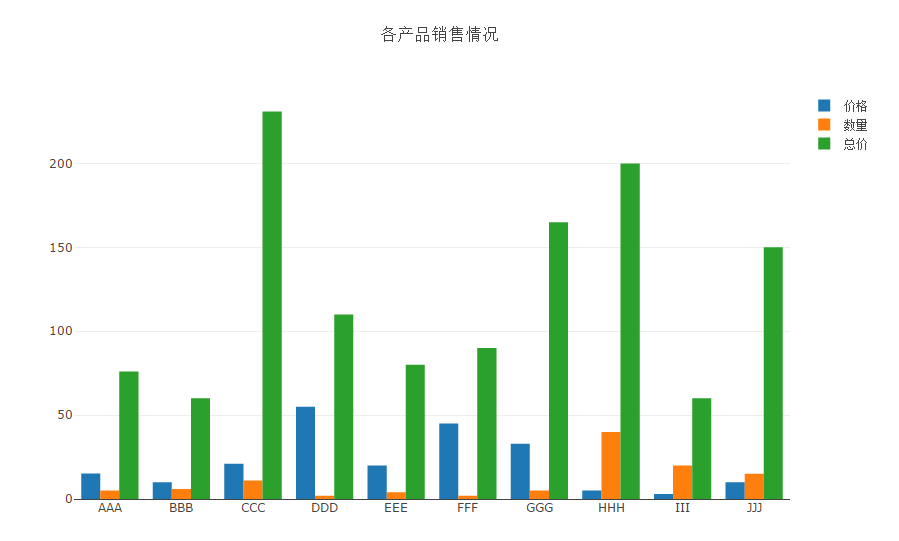展开全文• Python做数据分析绘制简单的条形图，解决简单的生活问题 ** 刚学数据分析不久，下面牛刀小试一次。 题目：为了对某一产品进行合理定价，我们对此类商品进行了试销实验，价格与需求量数据如下。利用图表分析规律。 ...
**
用Python做数据分析绘制简单的条形图，解决简单的生活问题
**
刚学数据分析不久，下面牛刀小试一次。
题目：为了对某一产品进行合理定价，我们对此类商品进行了试销实验，价格与需求量数据如下。利用图表分析规律。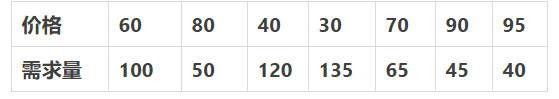需from matplotlib import pyplot as plt
import matplotlib
font = {
‘family’:‘SimHei’,
‘weight’:‘bold’,
‘size’:12
}
matplotlib.rc(“font”, **font)
plt.figure(figsize=(10,7))
price = [60,80,40,30,70,90,95]
sales = [100,50,120,135,65,45,40]
width = 0.3
Q = []
for i in range(0,len(price)):
Q.append(((price[i]*sales[i])*2)/100)
po_l = [i-width/2 for i in range(len(price))]
po_r = [i+width/2 for i in range(len(price))]
plt.bar(po_l,sales,width=width,label=‘销售量’,color=‘r’)
plt.bar(po_r,Q,width=width,label=‘赚取利润’,color=‘g’)
x_1 = [f"{i}元/件" for i in price]
y_t = range(0,140,5)
y_1 = [f"{i}万件" for i in y_t]
plt.xticks(range(len(price)),x_1,rotation=0,color=‘g’)
plt.yticks(y_t,y_1,color=‘g’,rotation=0)
plt.xlabel(‘销售价格’,color=‘b’)
plt.ylabel(‘销售量’,color=‘b’)
plt.title(‘产品销售利润分析’,color=‘r’)
plt.legend()
plt.show()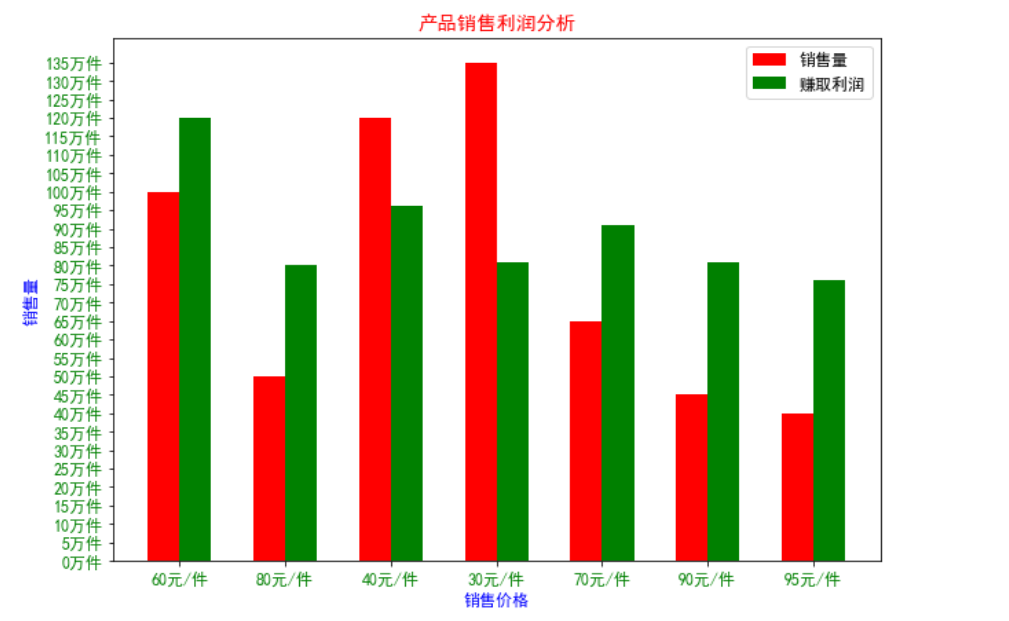求分析：要计算不同定价条件下的利润，只需要找出不同定价条件下的销售量即可，无需知道变化情况，只要能直观地反映出结果就行，因此选择条形统计图。代码和结果如下：
注意事项，设计刻度标签与绘制图形，二者井水不犯河水，因此，设计标签不需要严格要求横纵坐标一一对应。


展开全文python matplotlib
• 1、数据分组–>频数分布表 ...至于为什么要画水平条形图，当类别太多，使用水平条形图比较简洁，个人看法。下面给出代码，就不解释代码含义了。 def data_count(dataa, r1, r2, step): r = pd.DataFrame(np.random.ra
• 使用matplotlib展示数据的时候，当我们用到条形图的时候南面会遇到一些问题： 水平条形和竖直的条形问题： 解决方法就是更改下函数名，plt.bar()是默认竖直，而plt.barh()是默认水平，只不过坐标轴需要改动...标签 matplotlib
• 一个好用的php程序，php画一个数据图，可以方便的几句话就能画出你想要的折线图，条形图，饼形图等等，样式多多，例子多多
• 画图部分转自... 目录 1.处理数据 2.画条形图 导入包 from matplotlib import pyplot as plt import numpy as np from matplotlib.font_manager import FontProp...matplotlib
• 一、JSON 数据准备首先准备一份 JSON 数据，这份数据共有 3560 条内容，每条内容结构如下：本示例主要是以 tz（timezone 时区） 这一字段的值，分析这份数据里时区的分布情况。二、将 JSON 数据转换成 Python 字典...
• 动态条形图_练习数据_CSV格式，文章参考：《最简单方法！！python生成动态条形图》，欢迎大家下载体验~~
• echarts条形图横向显示数据且设置label属性Echarts条形图顶端显示数据默认不显示数据修改label属性控制数据显示位置 默认不显示数据 <!DOCTYPE html> <html style="height: 100%"> <head> <...
• # 导入模块import matplotlib.pyplot as pltimport numpy as npimport pandas as pd# 导入数据traffic_volume = {'Index': ['railway', 'green', 'water transport', 'air transport'],'Jan': [31058, 255802, 52244...
• 普通条形图、水平条形图 一、普通条形图 # @Author : Keep # @File : 普通条形图.py # 条形统计图统计电影排行前十的播放量 from matplotlib import pyplot as plt from matplotlib.font_manager import ...python
• 一、JSON 数据准备首先准备一份 JSON 数据，这份数据共有 3560 条内容，每条内容结构如下：本示例主要是以 tz（timezone 时区） 这一字段的值，分析这份数据里时区的分布情况。二、将 JSON 数据转换成 Python 字典...
• 项目描述 打开房天下数据表，其中包括了房天下网站从2009年1月到2010年12月的各大...按照2009年和2010年的第1、2、3、4季度共8个时间节点，画出各节点对应销售数量总和的条形图。（50分） 数据举例 实现代码 impo...可视化
• 之前Animated Bar Chart Race动态条形图感觉效果不太好，因为条块太短很多文字信息被遮挡了，于是找到了播放轴Play Axis插件，准备结合条形图插件在一次动态条形图。 原来Animated Bar Chart Race的动态效果...
• 分组条形图、堆叠条形图 一、分组条形图 # @Author : Keep # @File : 分组条形图.py from matplotlib import pyplot as plt from matplotlib.font_manager import FontProperties # 导入字体 font = ...python
• 花生米AJAX-UI系列之:基于JQUERY的投票结果对比条形图0.1版 特点： 可以对投票框的标题，每个投票项的名字，数据的单位，是否显示百分率等进行定义； 是否使用radio,checkbox等INPUT控件，也可自定义; 每项投票......